# Math Expanded Form Worksheets for Grade 4

The value of each digit in mathematics can be written in expanded forms. Writing numbers in the expanded form is to write a number by adding the value of its digit.  It helps understand a given number in a better way. It is very useful to split and present numbers in their units, tens, hundreds, and thousands form. The following worksheets consist of exercises to write numbers in their expanded form.

## Expanded Form Worksheets for KidsIt is an easy exercise to test one’s understanding of place values. He has to write the numbers in their expanded form as shown in the example above.This is the third worksheet. Fourth graders have to split the numbers by digit and use their place values to write these in the expanded forms. It is one of the most basic things we can learn to do with numbers.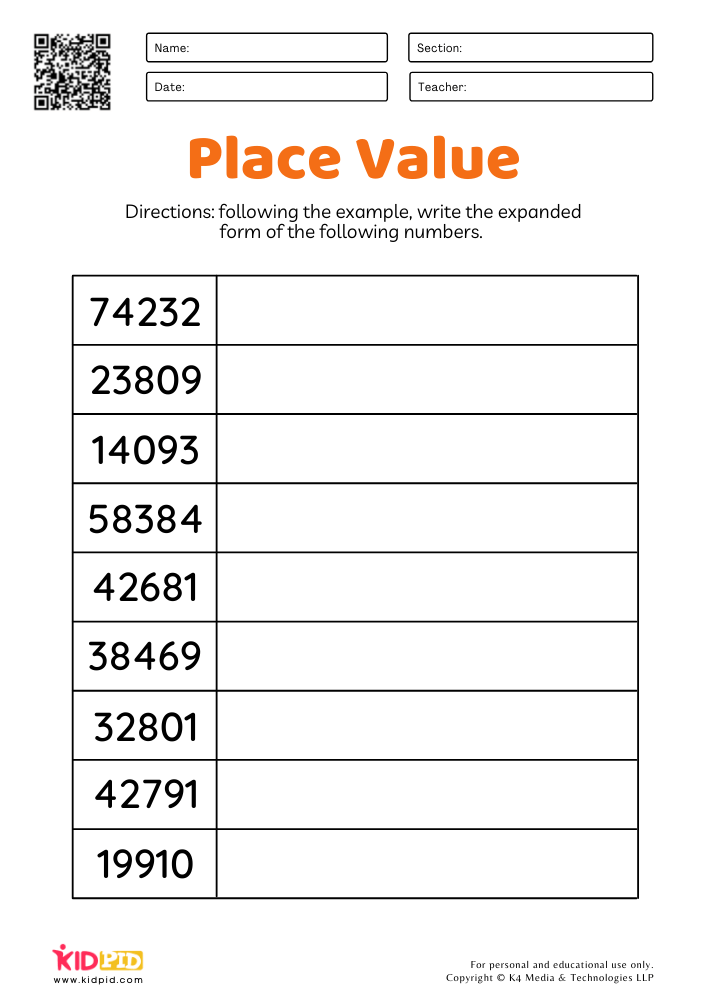The fourth worksheet gives more questions to work with. One can never have enough practice!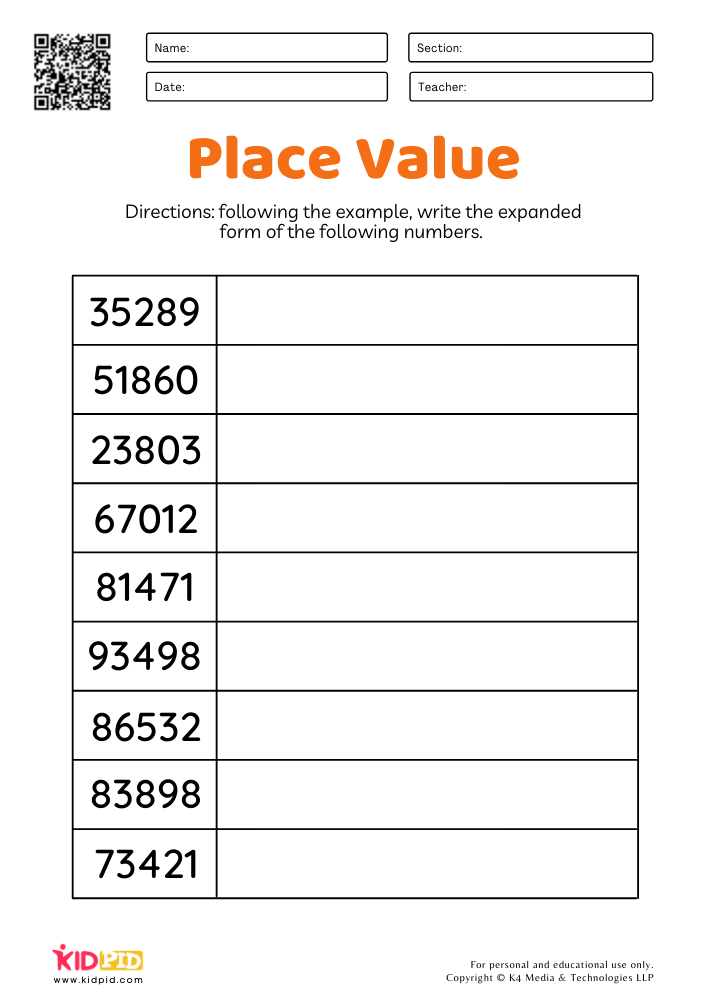This is the fifth worksheet that has nine more numbers to be written in their expanded form. Through regular practice of writing numbers in their expanded form one develops a better understanding of numbers.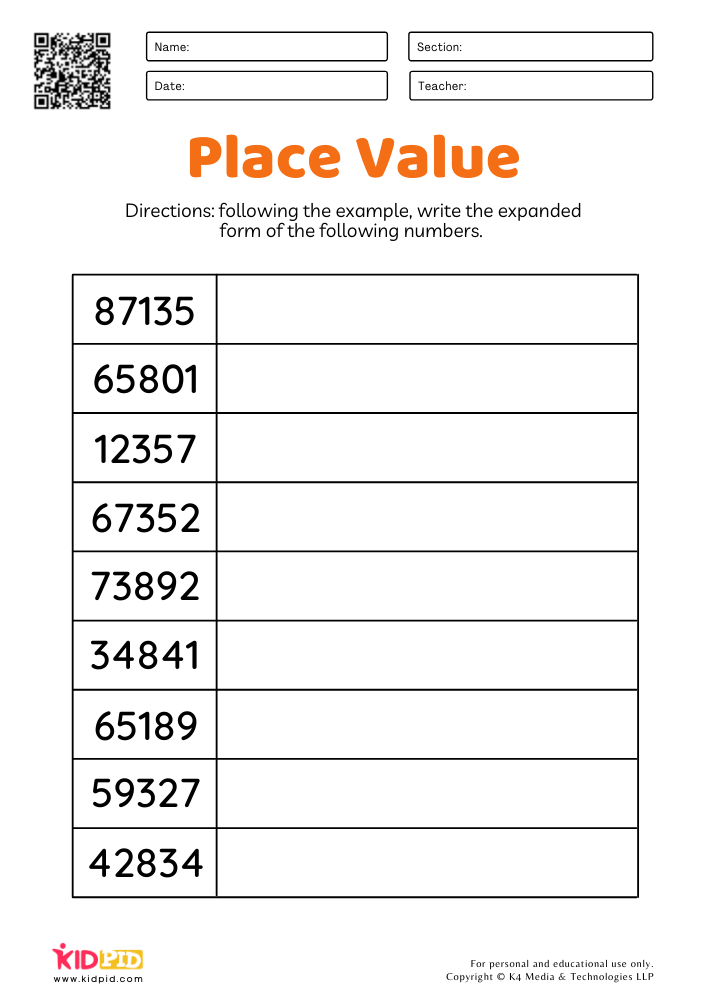Learning to write numbers in their expanded forms is essential as it allows students to read and write higher numbers properly. It is thus of great importance that one must practice until he grasps the concept.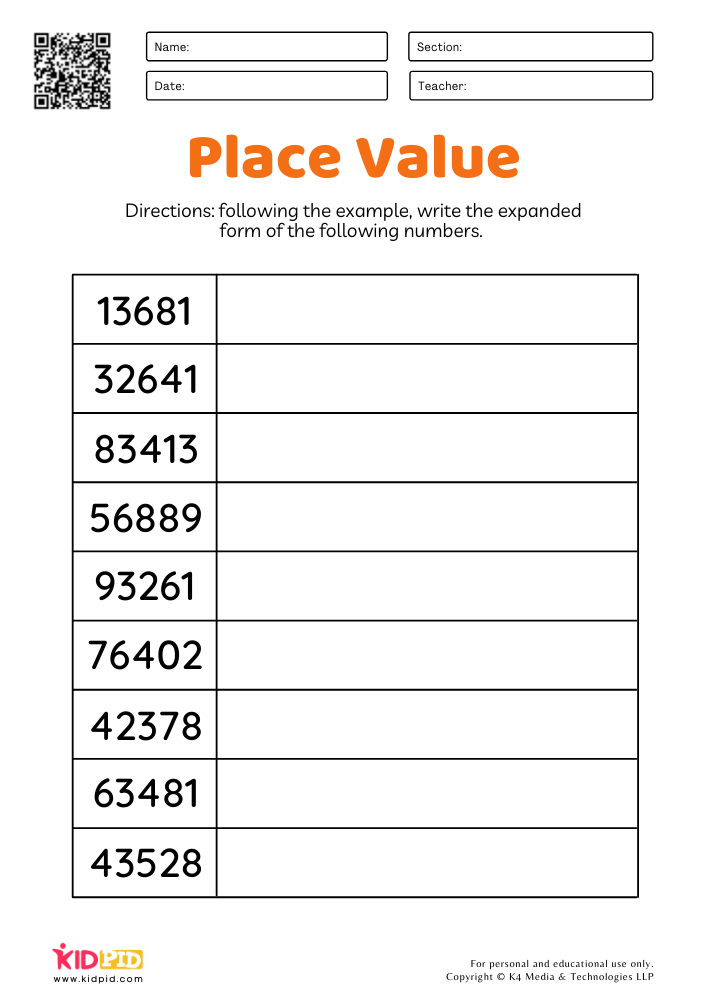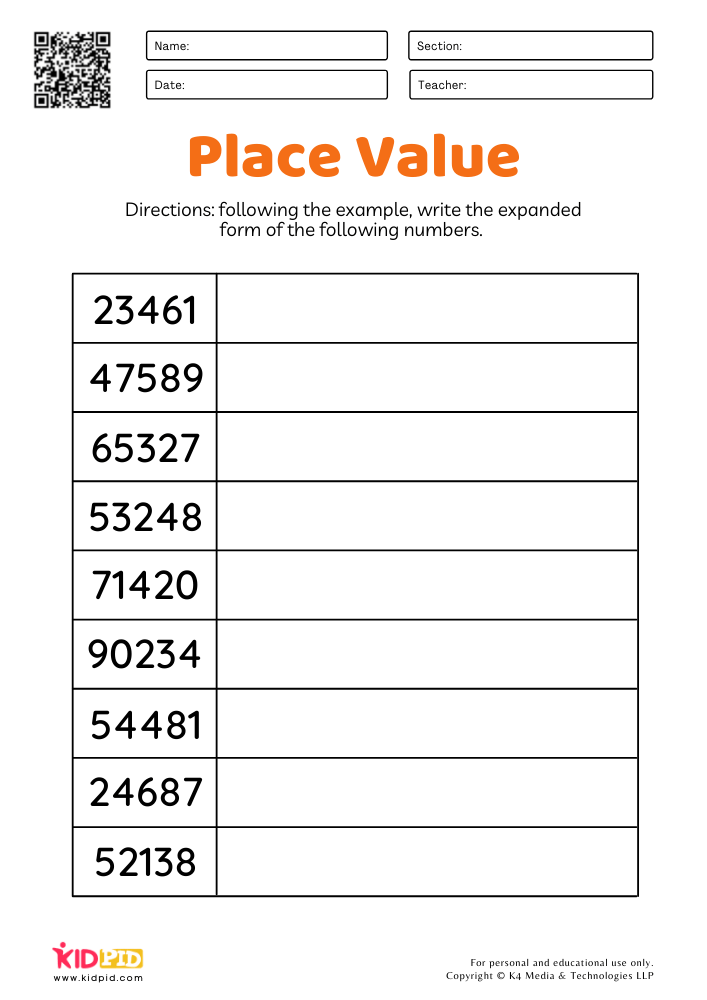It is a simple exercise to help children learn the fundamentals of numbers and place values in mathematics. Without the knowledge of place values and expanded forms, it is impossible for kids to perform arithmetic calculations.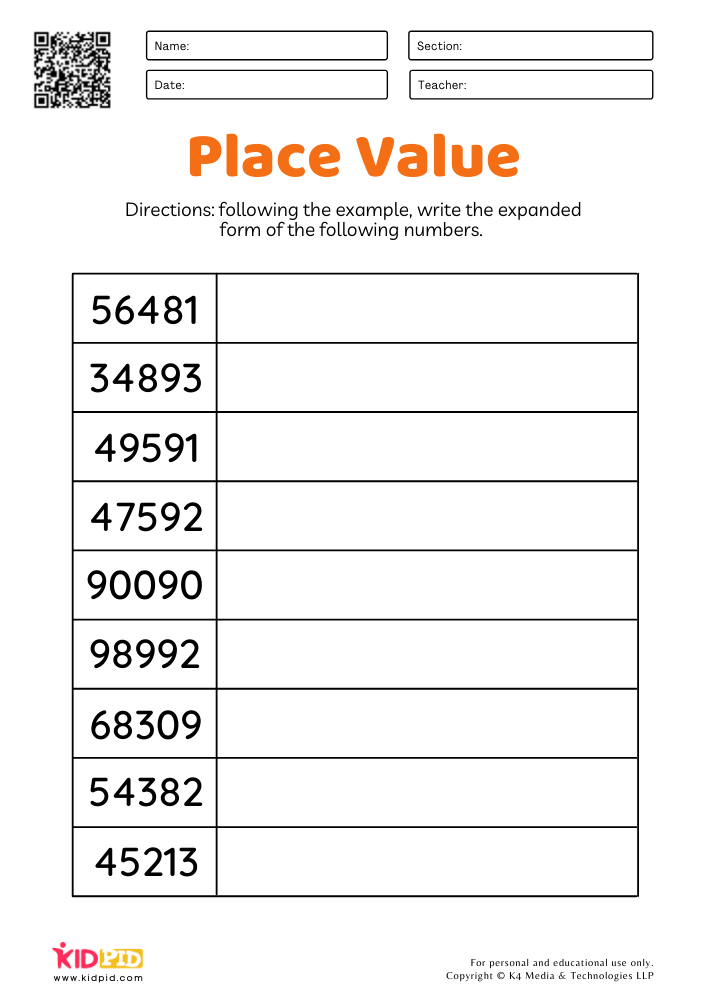All of these worksheets have numbers that can be expanded to the place value of ten thousand. It allows the children to practice and understand bigger numbers.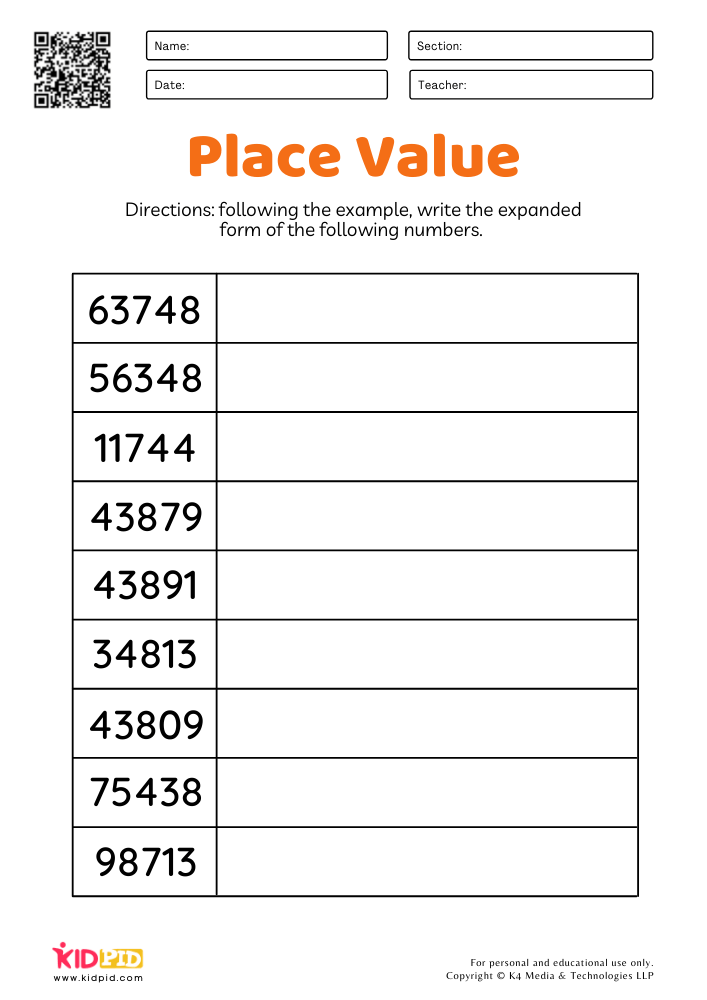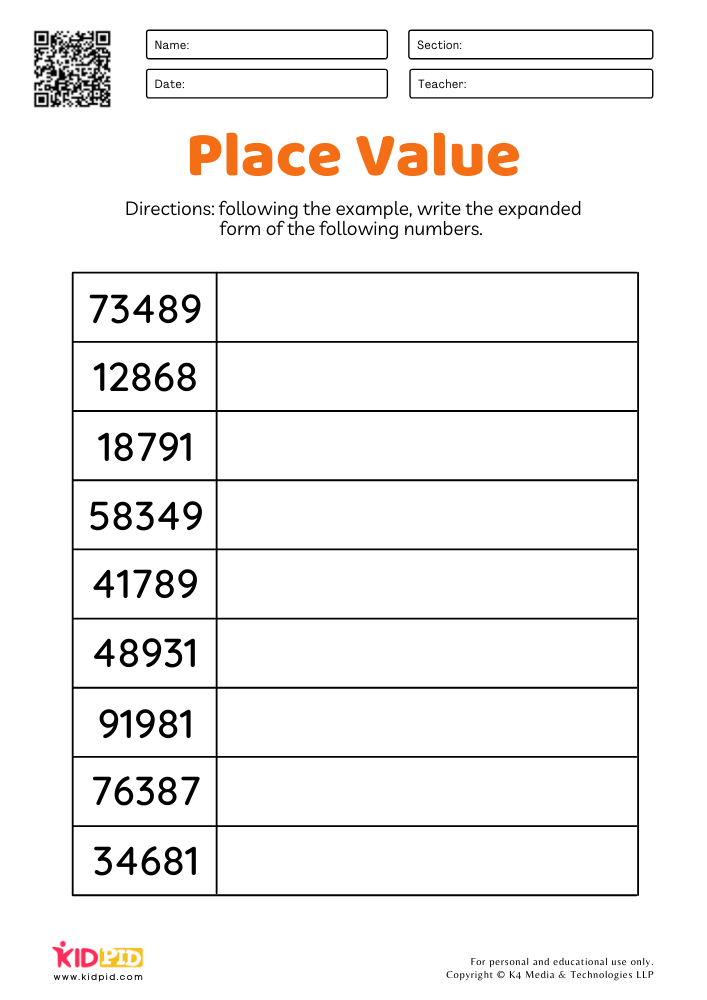The final sheet can be used to test the student’s ability to write the numbers in the expanded form. If he makes mistakes be sure to guide him and show him the right way to do it.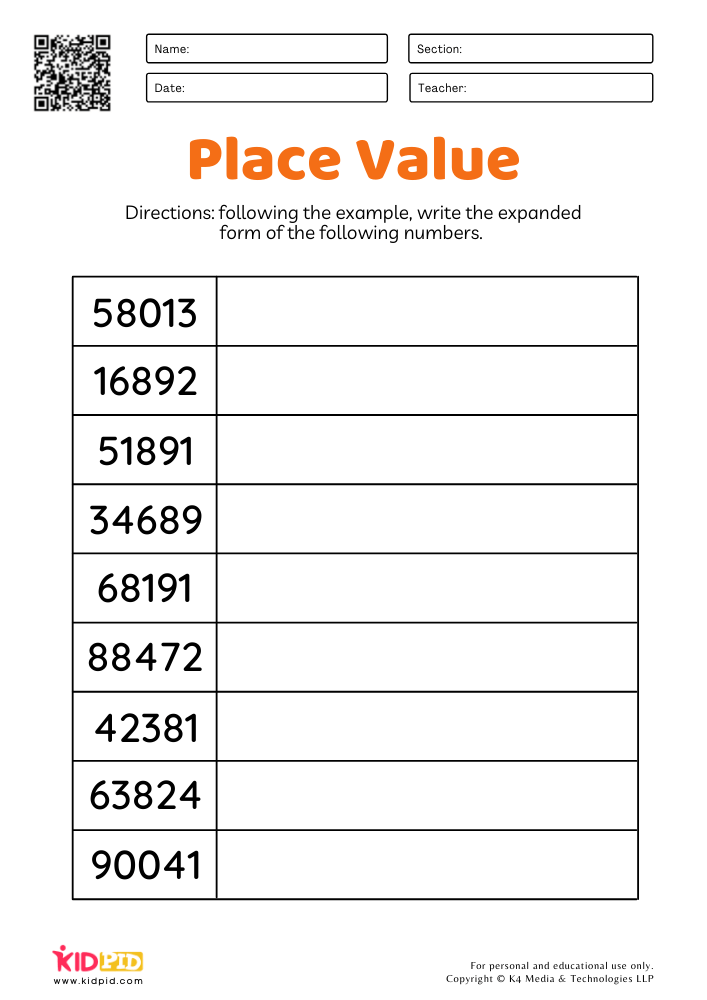There are several ways to write numbers in expanded form. These can be written in standard form using commas(,), or in expanded factors form,  word form, or in expanded exponential form. Writing the numbers as we have practiced in this worksheet is the beginner’s way to do it. Download the worksheets to teach your students how to write numbers in expanded forms.

## Place Value: 2-Digit Numbers Worksheets for Grade 1

The concept of Place Value is perhaps one of the most basic and a key fundamental concept in mathematics. The total understanding of the concept…

## Tens & Ones Part-Whole Model Worksheets for Grade 1

This worksheet consists of a total of ten sheets with exercises based on the part-whole model for identifying tens and ones. It is an introduction…

## Combining Tens and Ones Place Value Worksheets for Grade 1

In this edition, we have brought some worksheets that are going to help your child learn about combining numbers and work on Ones and Tens…

## Math Expanded Form Worksheets for Grade 1

To write a number in its expanded form is to write a number by adding the value of its digits. Place value charts are used…

## Combine tens & ones worksheets for Grade 1

In the abstract science of number and quantity – mathematics, addition is one of the four basic operations. The addition of two numbers results in…

+